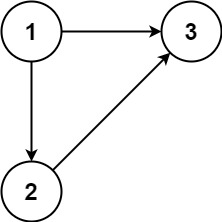## Algorithm

Problem Name: 685. Redundant Connection II

In this problem, a rooted tree is a directed graph such that, there is exactly one node (the root) for which all other nodes are descendants of this node, plus every node has exactly one parent, except for the root node which has no parents.

The given input is a directed graph that started as a rooted tree with `n` nodes (with distinct values from `1` to `n`), with one additional directed edge added. The added edge has two different vertices chosen from `1` to `n`, and was not an edge that already existed.

The resulting graph is given as a 2D-array of `edges`. Each element of `edges` is a pair `[ui, vi]` that represents a directed edge connecting nodes `ui` and `vi`, where `ui` is a parent of child `vi`.

Return an edge that can be removed so that the resulting graph is a rooted tree of `n` nodes. If there are multiple answers, return the answer that occurs last in the given 2D-array.

Example 1:```Input: edges = [[1,2],[1,3],[2,3]]
Output: [2,3]
```

Example 2:```Input: edges = [[1,2],[2,3],[3,4],[4,1],[1,5]]
Output: [4,1]
```

Constraints:

• `n == edges.length`
• `3 <= n <= 1000`
• `edges[i].length == 2`
• `1 <= ui, vi <= n`
• `ui != vi`

## Code Examples

### #1 Code Example with C++ Programming

```Code - C++ Programming```

``````
class Solution {
public:
vector findRedundantDirectedConnection(vector>& edges) {
vectorres;
unordered_mapparent;
unordered_map>g;
// c0: the last edge to form a circle
// c1, c2: parents of the node who has two parents
vectorc0, c1, c2;
int rootCandidate = 0, root = 0, count = 0;
for(auto x: edges){
int u = x, v = x;
if(parent[v] > 0){
c1 = x;
c2 = {parent[v], v};
}
if(!parent[u]){
rootCandidate++;
parent[u] = -1;
}
if(parent[v] == -1) rootCandidate--;
parent[v] = u;
if(rootCandidate == 0) c0 = x, rootCandidate = -1; // No valid root can be found, circle detected
g[u].push_back(v);
}
for(auto x: parent) if(x.second == -1) root = x.first;
int nodes = parent.size();
vectorvisited(nodes + 1);
return !root ? c0 : (!hasCircle(g, -1, root, c1, visited, count) && count == nodes) ? c1 : c2;
}

bool hasCircle(unordered_map>& g, int from, int root, vectorc, vector& visited, int& count){
if(from == c && root == c) return false;
if(visited[root]) return true;
count++;
visited[root] = 1;
bool foundCircle = false;
for(auto x: g[root]) foundCircle |= hasCircle(g, root, x, c, visited, count);
return foundCircle;
}
};
``````
Copy The Code &

Input

cmd
edges = [[1,2],[1,3],[2,3]]

Output

cmd
[2,3]

### #2 Code Example with Javascript Programming

```Code - Javascript Programming```

``````
const findRedundantDirectedConnection = function (edges) {
const parent = []
//detect circle
for (let i = 1; i <= edges.length; i++) {
parent[i] = i
}
let circleEdge, removedEdge, candidateEdge
for (let i = 0; i < edges.length; i++) {
const [u, v] = edges[i]
const pu = findParent(parent, u)
const pv = findParent(parent, v)
if (pv !== v) {
removedEdge = [u, v] // node with 2 parents
} else {
if (pv === pu) {
circleEdge = [u, v] // circle edge
}
parent[v] = pu
}
}
if (!removedEdge) {
return circleEdge
}
if (circleEdge) {
return edges.find((d) => d === removedEdge && d !== removedEdge)
} else {
return removedEdge
}
}
const findParent = function (parent, i) {
if (parent[i] !== i) {
parent[i] = findParent(parent, parent[i])
}
return parent[i]
}
``````
Copy The Code &

Input

cmd
edges = [[1,2],[1,3],[2,3]]

Output

cmd
[2,3]

### #3 Code Example with Python Programming

```Code - Python Programming```

``````
class Solution:
def findRedundantDirectedConnection(self, edges):
def root(i):
return parent[i] == i and i or root(parent[i])

parent, a, b, c =  * (len(edges) + 1), None, None, None
for i, edge in enumerate(edges):
if parent[edge]:
a, b, c, edges[i]= parent[edge], edge, edge, 0
else:
parent[edge] = edge

parent = [i for i in range(len(edges) + 1)]
for u, v in edges:
if u:
if root(u) == v:
return a and [a, c] or [u, v]
parent[v] = u
return [b, c]
``````
Copy The Code &

Input

cmd
edges = [[1,2],[2,3],[3,4],[4,1],[1,5]]

Output

cmd
[4,1]